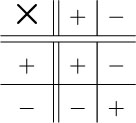Science, Maths & Technology

### Become an OU studentNumbers, units and arithmetic

Start this free course now. Just create an account and sign in. Enrol and complete the course for a free statement of participation or digital badge if available.

# 3.20 Multiplication rules

The rules for multiplying positive and negative numbers can be illustrated by the table below.

Multiplying a positive number by a positive number gives a positive answer.

Multiplying a negative number by a positive number gives a negative answer.

Multiplying a positive number by a negative number gives a negative answer.

Multiplying a negative number by a negative number gives a positive answer.The painting and the washing machine from Example 26 can be used again, to illustrate these rules.

## Example 29

A painting increases in value at £20 a year and a washing machine decreases in value at £20 a year. Each is worth £200 today.

• (a) How much will each be worth in four years’ time?

• (b) How much was each worth four years ago?

• (a) To find the value in four years’ time, in each case add the annual increase (which is negative in the case of the washing machine) four times. So the value of the painting will be

•    £200 + 4 × £20 = £200 + £80 = £280

• and the value of the washing machine will be

•    £200 + 4 × £20 = £200 − £80 = £120.

• (b) To find the value four years ago, subtract the annual increase from today's value four times. So the value of the painting four years ago was

•    £200 − 4 × £20 = £120

• and the value of the washing machine four years ago was

•    £200 − 4 × £20.

• But this is a matter of repeatedly subtracting a negative number, which is the same as repeatedly adding the corresponding positive number. So

•    £200 − 4 × £20 = £200 + 4 × £20 = £280.

## Try some yourself

### Activity 61

Evaluate each of the following and where possible give an example from everyday life to illustrate the calculation.

• (a) 4 × 3

• (b) 2 × 7

• (c) 3 × 5